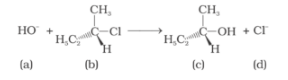# Which of the following statements are correctQuestion:

Which of the following statements are correct about the kinetics of this

reaction?(i) The rate of reaction depends on the concentration of only (b).

(ii) The rate of reaction depends on the concentration of both (a) and (b).

(iii) Molecularity of reaction is one.

(iv) Molecularity of reaction is two.

Solution:

Option (i) and (iii) are the answers.